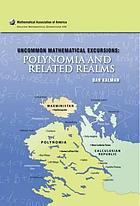Uncommon mathematical excursions : polynomia and related realms (Book, 2009) [WorldCat.org]Checking...

# Uncommon mathematical excursions : polynomia and related realms

Author: Dan Kalman Washington, D.C. : Mathematical Association of America, ©2009. Dolciani mathematical expositions, no. 35.Print book : EnglishView all editions and formats This textbook explores the extensions, digressions and supplementary topics are often ignored in tradition curriculum such as additional properties of polynomials, rational functions and exponentials. Derivatives without limits, constrained maxima and minima with Lagrangian functions, envelopes and asymptotes and generalized logarithms for exponential- linear equations are also explained--Annotation ©2009 Book News, Inc., Portland, OR.  Read more... (not yet rated) 0 with reviews - Be the first.

## Find a copy in the libraryFinding libraries that hold this item...

## Details

Material Type: Internet resource Book, Internet Resource Dan Kalman Find more information about: Dan Kalman 9780883853412 0883853418 290454936 xv, 265 pages : illustrations ; 26 cm I. The province of polynomia. Horner's form -- Polynomial potpourri -- Polynomila roots and coefficeints -- Solving polynomial equations -- II. Maxministan. Leveling with Lagrange: constrained maxima and minima with Lagrangian functions -- A maxmini miscellany -- Envelopes and the ladder problem -- Deflection on an ellipse -- III. The Calculusian Republic. A generalized logarithm for exponential-linear equations -- Envelopes and asymptotes -- Derivatives without limits -- Two Calculusian Miracles. Dolciani mathematical expositions, no. 35. Polynomia and related realms Dan Kalman.

### Abstract:

This textbook explores the extensions, digressions and supplementary topics are often ignored in tradition curriculum such as additional properties of polynomials, rational functions and exponentials. Derivatives without limits, constrained maxima and minima with Lagrangian functions, envelopes and asymptotes and generalized logarithms for exponential- linear equations are also explained--Annotation ©2009 Book News, Inc., Portland, OR.

## Reviews

User-contributed reviewsBe the first.

## Similar Items

### Related Subjects:(8)

Confirm this request

You may have already requested this item. Please select Ok if you would like to proceed with this request anyway.